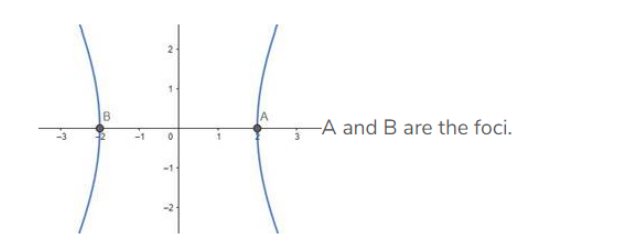# Find the equation of the hyperbola whose vertices are`
Question:

Find the equation of the hyperbola whose vertices are (±2, 0) and the eccentricity is 2.

Solution:

Given: Vertices are (±2, 0) and the eccentricity is 2

Need to find: The equation of the hyperbola.

Let, the equation of the hyperbola be:

$\frac{x^{2}}{a^{2}}-\frac{y^{2}}{b^{2}}=1$

Vertices are $(\pm 2,0)$, that means, $a=2$

And also given, the eccentricity, $e=2$We know that, $e=\sqrt{1+\frac{b^{2}}{a^{2}}}$

Therefore,

$\Rightarrow \sqrt{1+\frac{b^{2}}{a^{2}}}=2$

$\Rightarrow 1+\frac{\mathrm{b}^{2}}{\mathrm{a}^{2}}=4$ [Squaring both sides]

$\Rightarrow \frac{\mathrm{b}^{2}}{\mathrm{a}^{2}}=3$

$\Rightarrow \mathrm{b}^{2}=3 \mathrm{a}^{2}=3 \times 4=12[$ As $\mathrm{a}=2]$

So, the equation of the hyperbola is,

$\frac{x^{2}}{a^{2}}-\frac{y^{2}}{b^{2}}=1 \Rightarrow \frac{x^{2}}{4}-\frac{y^{2}}{12}=1[$ Answer $]$